plot 订阅
matlab中二维线画图函数。

plot(y)等

plot

matlab
plot语法
plot函数的基本调用格式为：1、plot(y)当y为向量时，是以y的分量为纵坐标，以元素序号为横坐标，用直线依次连接数据点，绘制曲线。若y为实矩阵，则按列绘制每列对应的曲线。2、plot(x,y)若y和x为同维向量，则以x为横坐标,y为纵坐标绘制连线图。若x是向量，y是行数或列数与x长度相等的矩阵，则绘制多条不同色彩的连线图，x被作为这些曲线的共同横坐标。若x和y为同型矩阵，则以x,y对应元素分别绘制曲线，曲线条数等于矩阵列数。3、plot(x1,y1,x2,y2,……)在此格式中，每对x,y必须符合plot(x,y)中的要求，不同对之间没有影响，命令将对每一对x,y绘制曲线。以上三种格式中的x,y都可以是表达式。plot是绘制一维曲线的基本函数，但在使用此函数之前，须先定义曲线上每一点的x以及y坐标。

• ## matlab中plot函数用法

万次阅读 多人点赞 2019-03-06 14:41:15
1.简单的2维直线图 : plot(x,y) 同一坐标显示n条线：plot(x,y1,x,y2，…) x = 0:pi/10:2*pi; y = sin(x); figure; hold on; plot(x,y) 2.plot(X):X是矩阵，表示矩阵的每一行都画一条线，将显示n条线。 X=rand(3,3)...
线条、颜色等参数：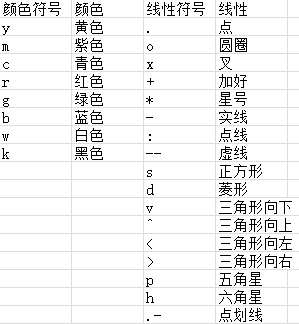1.简单的2维直线图 : plot(x,y)
同一坐标显示n条线：plot(x,y1,x,y2，…)
x = 0:pi/10:2*pi;
y = sin(x);
figure;
hold on;
plot(x,y)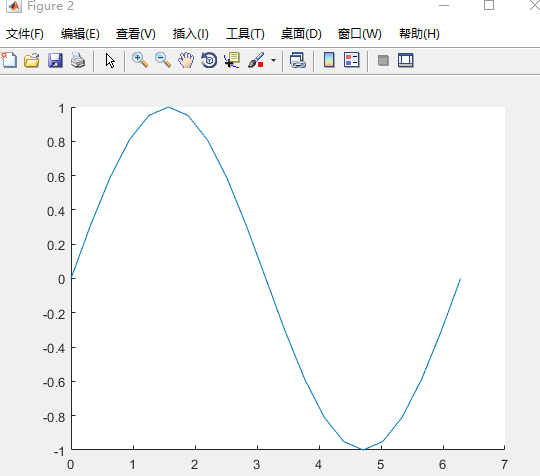2.plot(X):X是矩阵，表示矩阵的每一行都画一条线，将显示n条线。
X=rand(3,3); %随机生成3*3矩阵
figure;
hold on;
plot(X)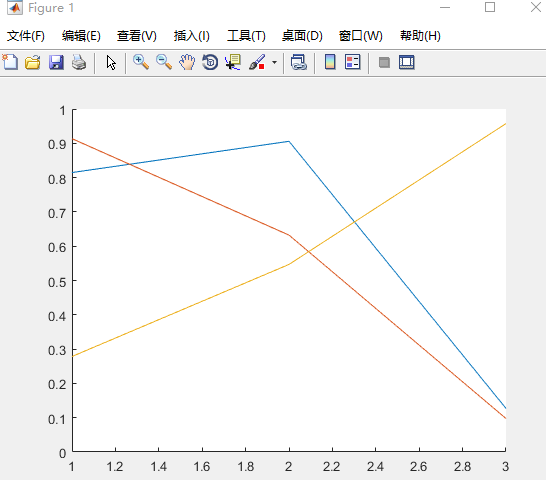3.画带形状的图
plot(X(pos, 1), X(pos, 2), 'k+','LineWidth', 2, 'MarkerSize', 7);
plot(X(neg, 1), X(neg, 2), 'ko', 'MarkerFaceColor', 'y','MarkerSize', 7);

X(pos, 1), X(pos, 2)表示横纵坐标
'k+'表示线性为加号型、黑色
LineWidth', 2表示线宽为2
'MarkerSize', 7表示形状大小为7
'MarkerFaceColor', 'y'表示形状填充颜色为黄色
另：MarkerEdgeColor','b'表示方框边缘颜色为蓝色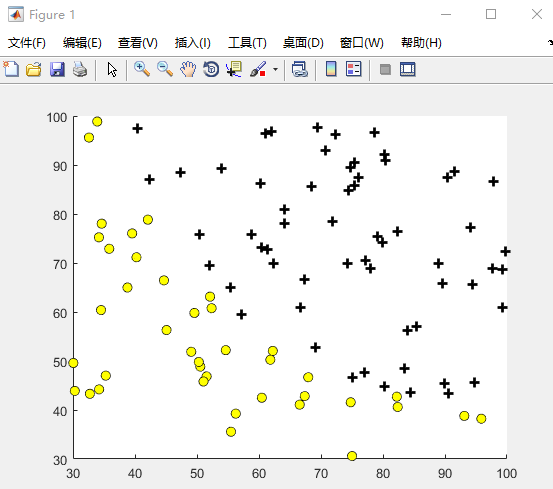展开全文• ## matlabplot画图详解

千次阅读 2015-09-16 11:09:33
clear clc x=[0.1 0.5 1 5 10 50 100 500 1000]; ...y1=[29.22 29.03 28.97 28.91 28.93 28.92 28.92 28.92 28.92];...y2=[37.18 37.20 37.21 37.22 37.22 37.22 37.22 37.22 37.22];...y3=[20.38 19.95 19.95 19
clear
clc
x=[0.1 0.5 1 5 10 50 100 500 1000];
y1=[29.22 29.03 28.97 28.91 28.93 28.92 28.92 28.92 28.92];
y2=[37.18 37.20 37.21 37.22 37.22 37.22 37.22 37.22 37.22];
y3=[20.38 19.95 19.95 19.89 19.89 19.89 19.89 19.89 19.89];
plot(x,y1,'k-s','LineWidth',2,'MarkerEdgeColor','k','MarkerSize',8);
%LineWidth控制线条宽度，k控制线条颜色，-控制线条是直线，s控制线条颜色，MarkerEdgeColor控制点边缘的颜色，MarkerSize控
%制点的大小
hold on;
plot(x,y2,'r-d','LineWidth',2,'MarkerEdgeColor','r','MarkerSize',8);
hold on;
plot(x,y3,'b-x','LineWidth',2,'MarkerEdgeColor','b','MarkerSize',8);
legend('sin','cos','tan',0);
%legend控制每条曲线的显示信息，0控制显示信息的位置
xlabel('\lambda','FontName','Times','Fontsize',14);
ylabel('Accuracy(%)','FontName','Times','Fontsize',14);
%xlabel和ylabel控制坐标的显示，FontName表示字体：Times，Fontsize控制字的大小，注意一定要在画图后执行，否则图形显示不%出来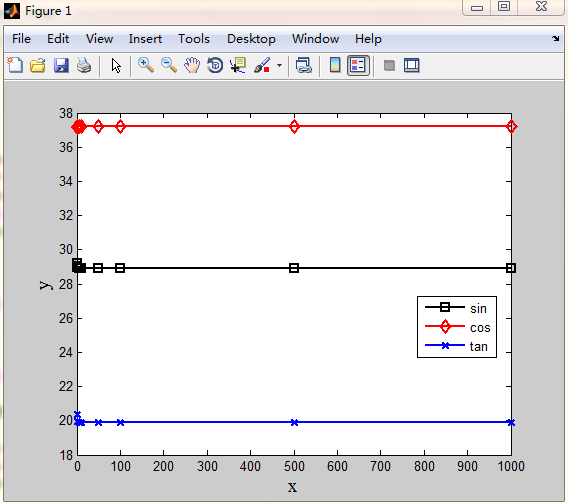展开全文• MATLAB导入Excel数据并用plot函数绘图 第一次写博客，心里有点小激动！写这一篇博客的目的是帮助像我一样刚入门的小白，因为昨天查了相关博客，但是发现和我想找的还是比较少的，所以特此写一篇来总结一下我摸索出来...
MATLAB导入Excel数据并用plot函数绘图
第一次写博客，心里有点小激动！写这一篇博客的目的是帮助像我一样刚入门的小白，因为昨天查了相关博客，但是发现和我想找的还是比较少的，所以特此写一篇来总结一下我摸索出来的经验。
第一步：打开matlab并找导入数据这一项第二步：点击并找到需要导入的excel文件第三步：导入并选中需要导入工作区的数据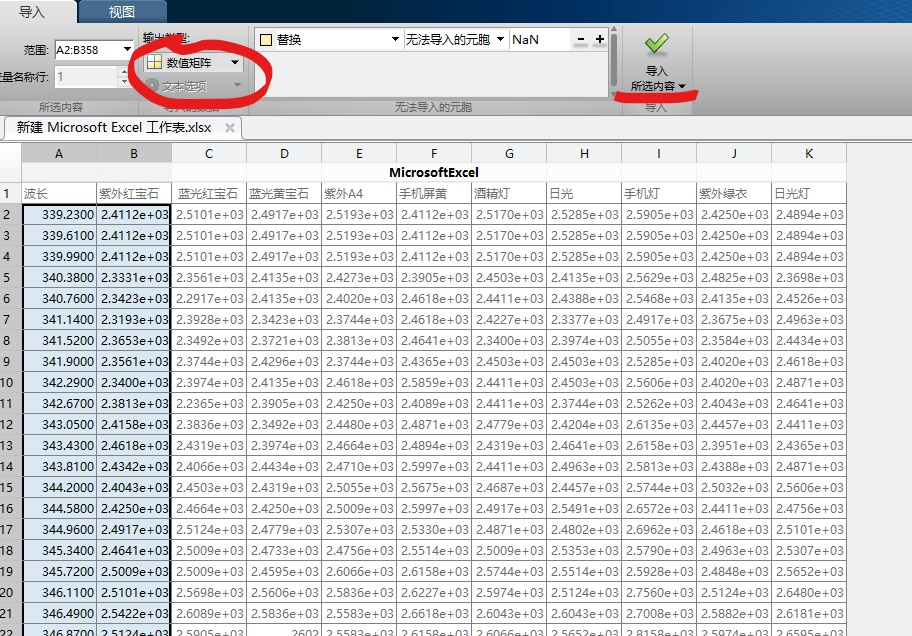第四步：用plot绘图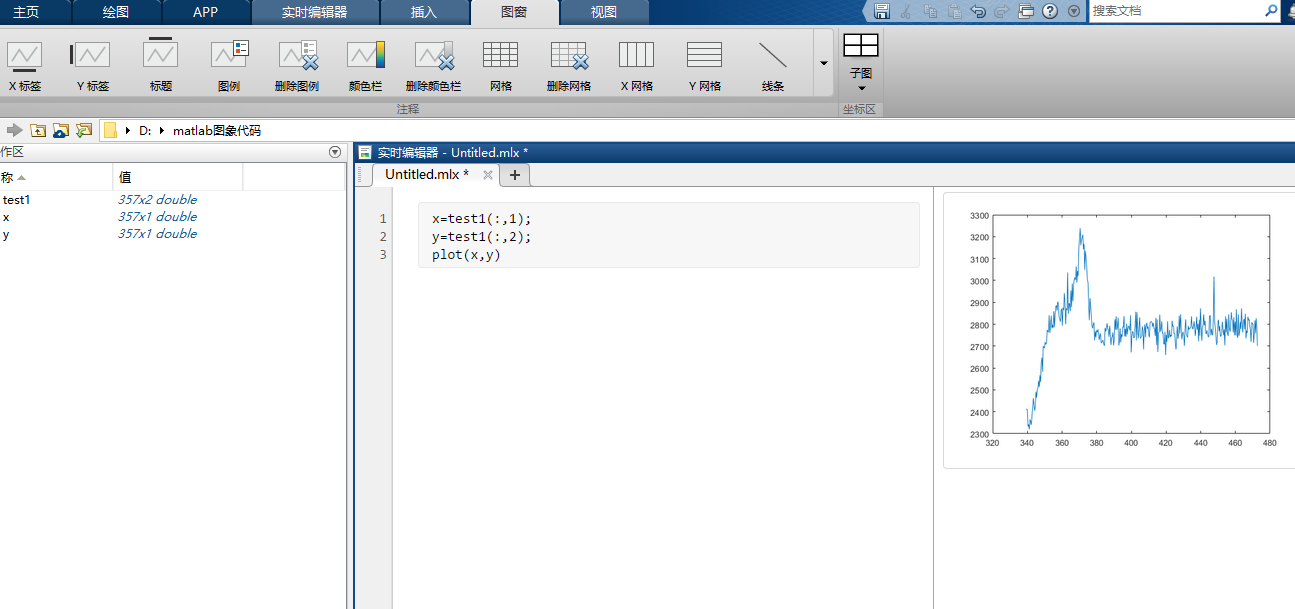其他关于matlab制图的知识可自行查看官方文档，在此不做介绍。
如有错误，还望各位指出！


展开全文Excel
• 由于粗心，没有看matlab plot的资料，把 plot(x,y,..)中 x 和 y 的顺序弄反了，调试了一段时间才发现这个问题，现记录一下，防止再犯。  在 Matlab 中，plot( x, y , ...)中，x 是横坐标，也就是矩阵中列号，y是纵...
    由于粗心，没有看matlab plot的资料，把 plot(x,y,..)中 x 和 y 的顺序弄反了，调试了一段时间才发现这个问题，现记录一下，防止再犯。
在 Matlab 中，plot( x, y , ...)中，x 是横坐标，也就是矩阵中列号，y是纵坐标，也就是矩阵中行号。
魔鬼往往隐藏于细节之中，小心为好。

展开全文• ## Matlabplot

千次阅读 2010-05-21 14:43:00
二维曲线绘图语法plot(Y)plot(X1,Y1,...)plot(X1,Y1,LineSpec,...)plot(...,PropertyName,PropertyValue,...)plot(axes_handle,...)h = plot(...)hlines = plot(v6,...)描述plot(Y)如果Y是m×n的数组，以1:m为X横...c
• ## matlabplot函数详解

万次阅读 多人点赞 2018-04-28 17:04:08
matlab中，plot函数用来绘制二维图像。1.plot默认格式 plot(x,y)这种格式中，若x,y是向量，则它们必须具有相同的长度。函数将以x为横轴，绘制y。 若x,y都是矩阵，则它们必须具有相同的尺寸，plot函数将针对x的各...
• 本部分介绍MATLAB的图形窗口和二维图形的绘制： 一.二维绘图： 1.plot绘图命令： plot哈桑农户是最基本最常用的绘图命令，执行plot命令，系统会自动创建一个新的图形窗口， plot函数有以下几种调用格式： 1）...
• ## MATLAB画函数图像

万次阅读 多人点赞 2019-04-19 15:56:34
1 画图基础 （1）一元一次函数 x=0:0.1:1; y=x; plot(x,y); %图像见下图1 图1 ...
• MATLABplot函数常常被用于绘制各种二维图像，其用法也是多种多样，本文仅介绍plot函数的基本用法——使用plot函数绘制二维点图和线图。plot函数的一般调用形式如下： plot(X, Y, LineSpec) 其中X由所有输入点坐标...
• 前段时间用SVR调参，需要对C的权重进行修改，所以上网搜...plt.plot(X, y, 'bo', fillstyle='none') plt.plot(X, result1, 'r.') 红色 plt.plot(X, result2, 'g.') 绿色 plt.plot(X, result3, 'c.') 亮蓝 plt.plot(...
• ## MATLAB中plot函数的用法

万次阅读 多人点赞 2018-04-23 15:47:04
使用plot绘制二维图像本文转自http://blog.sina.com.cn/s/blog_d8f783c90102woqb.html以及https://blog.csdn.net/alvern_zhang/article/details/51153058MATLABplot函数常常被用于绘制各种二维图像，其用法也是...
• 一、Matlab绘图中用到的直线属性包括： （1）LineStyle：线形 （2）LineWidth：线宽 （3）Color：颜色 （4）MarkerType：标记点的形状 （5）MarkerSize：标记点的大小 （6）MarkerFaceColor：标记...
• ## plt.plot()的使用方法以及参数介绍

万次阅读 多人点赞 2019-05-21 09:07:48
偶然的一次操作，对plt.plot()中，仅仅传入了一个列表，然后逻辑就和预想的出现了偏差。 plt.plot() plt.plot() 参数介绍： x, y : array-like or scalar The horizontal / vertical coordinates of the data ...matplotlib python pyplot
• MATLAB中提供的线型属性有：   线型 说明 标记符 说明 颜色 说明 - 实线(默认) + 加号符 r 红色 -- 双划线 o 空心...
• plot(X,Y) 绘制单条曲线 x = 0:pi/100:2*pi; //表示x取0到2*pi之间的值，每个值之间间隔pi/100 y = sin(x); plot(x,y); plot(x,y1,x,y2) 绘制多条曲线 x = linspace(-2*pi, 2*pi);//x取值范围为-2*pi到2*pi...
• ## matlab之plot、fplot、ezplot函数

万次阅读 多人点赞 2016-06-03 13:31:10
一、plot函数 函数plot 是绘制二维图形的最基本函数，它是针对向量或矩阵的列来绘制曲线的。也就是说，使用plot 函数之前，必须首先定义好曲线上每一点的x 及y 坐标，常用格式为： （1）plot(x) 当x 为一向量时，...fplot
• ## MATLABplot绘图参考

千次阅读 2012-10-26 21:52:03
为了给一些同学带来方便，一下给出一个plot的例子，包括的比较全了。这个非常基础。一下是我某次写论文的时候用到的其中一个简单的绘图例子，有兴趣的可以参考一下： close all; c_psnr=[28.31 31.37 33.5
• MATLAB绘制二维曲线-plot函数plot函数的基本用法：plot函数最简单的调用格式：x，y为矩阵时含多个输入参数的plot函数plot函数的选项 plot函数的基本用法： plot(x,y) %xy分别用于存储x坐标和y坐标数据，通常x,y为...绘制二维曲线
• Matlab_plot的详细用法 Matlab画图说明
• ## matlab 冲激函数表示

万次阅读 2017-01-09 14:37:52
matlab中有一个dirc（）函数，用于δ函数的表示。 想画出冲激函数图像，可以利用如下代码：clc; clear all; x=0:200; %x轴范围 y=dirac(x-50); %x=50处有δ函数，即δ(x-50) y=1.5*sign(y); %改变幅度 plot(x,y); ...
• ## MATLAB中subplot的使用

万次阅读 2018-09-22 23:37:20
MATLAB中subplot函数的使用 subplot(1,2,1); plot(x,s1); subplot(1,2,2); plot(x,s2);subplot
• ## Matlabplot画图的用法

千次阅读 2014-05-05 22:43:08
1、设置图线宽度 set( haxis,'LineWidth', 1.0 ); 'LineWidth'就是axis的线宽度属性，其值默认为0.5，这里可以改成1.0了。   2、调整坐标轴上下限 set( haxis, 'XLim', [ 2 20 ] ); set( haxis, 'YLim', [ 2 ...
• ## matlabplot fplot函数

千次阅读 2020-01-17 17:49:07
plot （1）plot(x,y) 例子：折线 >> x=[2,4,8]; >> y=[5,3,9]; >> plot(x,y) （2）plot(x) 横坐标是下标，纵坐标是值 >> x=[2,4,8]; >>> plot(x) （3）plot（x,y）函数参数的...
• ## matlabplot 相关

千次阅读 2018-07-10 10:20:40
1. 线型，颜色，符号线型说明标记符说明颜色说明-实线(默认)+加号符r红色--双划线o空心圆g绿色:虚线*星号b蓝色:.... 标注，标题，坐标轴标注figure,plot(a2(1:26,2),a2(1:26,3),'k.'),hold on, plot(a2...
• plotMATLAB中的二维线画图函数 包括以下语法(摘自帮助文档）： plot(x,y) plot(x,y,linespec) plot(x1,y1,...,xn,yn) plot(x1,y1,linespec1,...,xn,yn,linespecn) plot(y) plot(y,linespec) plot(_,name,...
• 坐标轴标注 https://jingyan.baidu.com/article/da1091fb1770a1027849d692.html
• matlabplot函数画线时有很多的颜色和类型可以选择，下面列举一些。 常用的3+4+1=8种颜色：（速记：RGB、CYMK、W，即两种色彩空间模型+白色） 'r' 红色 'g' 绿色 'b' 蓝色 'c' 青色 'y' 黄色 'm' 品红 ...
• ## matlabplot绘图

千次阅读 2015-09-23 15:16:19
看到很多论文做的图都很漂亮，特此简单学习一下，文章后面有很多软件要学习，还有待进一步认真学习，matlab只是一个菜鸟级别的水平： function [ ] = Overhead_rate_step_number( ) %step number step=[1 2 3 4]; %...
• ## matlabplot 绘图

千次阅读 2011-12-09 14:25:27
plot函数用法  ...本节介绍MATLAB 的两种基本绘图功能：二维平面图形和三维立体图形。 5.1 二维平面图形 5.1.1 基本图形函数 plot 是绘制二维图形的最基本函数，它是针对向量或矩阵的列来绘制曲线的。string 网络
• https://ww2.mathworks.cn/products/matlab/plot-gallery.html...